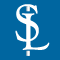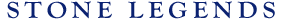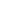HOMEPRODUCTSIDEA CENTERSERVICESTECHNICALABOUT USHello Guest Login  |  Become Member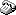800-398-1199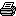214-398-1293MapEmail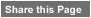Click to Share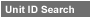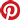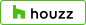Select which variables you would like to search by.Type DescriptionPrimary ViewPrimary ModifierUnit NameView Definitions

View Definitions

View DefinitionsX-Dim Y-Dim Z-Dim XX-Dim YY-Dim ZZ-Dim R-Dim D-Dim P-Dim
 Click column heading to re-sort results list.Product Name Primary View Modifier Pri Dimx  y  z Alt Dimxx  yy  zz Alt2 Dimr  d  p E\$timated Thumbnail Belleville1A Extrados1 Chamfer1 Stair Straight X = 36" Y = 12"Z = 9-1/2" XX = n/a YY = n/aZZ = n/a R = n/a D = n/aP = n/a \$ 82.00Caruth Straight Stairs Stair Straight X = 27-1/8" Y = 12"Z = 9-1/2" XX = n/a YY = n/aZZ = n/a R = n/a D = n/aP = n/a \$ 78.00Florentine Grand Stair Stair Grand Stair FinishedEnd X = 192" Y = 144-1/2"Z = 27-3/4" XX = 283" YY = n/aZZ = 41-5/8" R = 185-3/4" D = n/aP = n/a \$ 999999.00Florentine Straight Stairs Stair Straight X = 38-7/8" Y = 12"Z = 9" XX = n/a YY = n/aZZ = n/a R = n/a D = n/aP = n/a \$ 145.00Jefferson1 Straight Stairs Stair Straight X = 32-1/8" Y = 12"Z = 10-1/2" XX = n/a YY = n/aZZ = n/a R = n/a D = n/aP = n/a \$ 85.00Jefferson2 Straight Stairs Stair Straight X = 36-5/8" Y = 12"Z = 10-1/2" XX = n/a YY = n/aZZ = n/a R = n/a D = n/aP = n/a \$ 85.00Kendal Connelly1 Cove1 Stair Helical NewelPier X = 41-5/8" Y = 62-13/16"Z = 10-1/2" XX = n/a YY = n/aZZ = n/a R = 55-5/16" D = n/aP = n/a \$ 2789.00Kendal Straight Stairs Stair Straight X = 36" Y = 12"Z = 10-1/2" XX = n/a YY = n/aZZ = n/a R = n/a D = n/aP = n/a \$ 88.00Kinnard1 Straight Stairs Stair Straight X = 42-3/8" Y = 12"Z = 10-1/2" XX = n/a YY = n/aZZ = n/a R = n/a D = n/aP = n/a \$ 95.00Kinnard1Connely1 Cove1 Stair Helical NewelPier X = 54-5/8" Y = 72"Z = 10-1/2" XX = n/a YY = n/aZZ = n/a R = 96" D = n/aP = n/a \$ 2577.55Memorial Straight Stairs Stair Straight X = 36" Y = 12"Z = 9" XX = n/a YY = n/aZZ = n/a R = n/a D = n/aP = n/a \$ 90.00Noble Straight Stairs Stair Straight X = 36" Y = 12"Z = 9" XX = n/a YY = n/aZZ = n/a R = n/a D = n/aP = n/a \$ 99.00Oakley Stairs Stair Straight X = 36" Y = 12"Z = 8-7/8" XX = n/a YY = n/aZZ = n/a R = n/a D = n/aP = n/a \$ 88.32Otterwood Binelli Single Stair Spiral NewelPier X = 65-13/16" Y = 48"Z = 163-1/2" XX = n/a YY = n/aZZ = n/a R = 64-3/4" D = n/aP = n/a \$ 5028.11Otterwood Extrados1 Chamfer1 Stair Helical NewelPier X = 41-1/8" Y = 108"Z = 9-1/2" XX = n/a YY = n/aZZ = 9" R = 243-3/4" D = n/aP = n/a \$ 6285.46Otterwood Extrados1 Chamfer1 Stair Helical FinishedEnd X = 36" Y = 77-15/16"Z = 17-3/4" XX = n/a YY = n/aZZ = n/a R = 77-15/16" D = n/aP = n/a \$ 1600.00Otterwood Grand Stair 3 Stair Grand Stair NewelPier X = 41-5/8" Y = 80"Z = 108" XX = n/a YY = n/aZZ = n/a R = 124" D = n/aP = n/a \$ 13869.17Otterwood Grand Stair 4 Stair Grand Stair NewelPier X = 41-5/8" Y = 49"Z = 128-1/4" XX = n/a YY = n/aZZ = n/a R = 212" D = n/aP = n/a \$ 14535.29Otterwood Grand Stair 5 Stair Spiral NewelPier X = 41-5/8" Y = 54-3/4"Z = 102" XX = n/a YY = n/aZZ = n/a R = 134" D = n/aP = n/a \$ 13594.01Otterwood Grand Stair 6 Stair Grand Stair X = 207-7/8" Y = n/aZ = 36-5/8" XX = n/a YY = n/aZZ = n/a R = 96" D = n/aP = n/a \$ 999999.00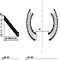Otterwood Helical 1 Ramp Stair Helical Helical X = 72" Y = 3-3/4"Z = 63" XX = n/a YY = n/aZZ = n/a R = n/a D = n/aP = n/a \$ 1092.93Otterwood Helical 13 Stair Grand Stair NewelPier X = 41-5/8" Y = 140-9/16"Z = 9-1/2" XX = n/a YY = n/aZZ = n/a R = 374-5/8" D = n/aP = n/a \$ 3415.00Otterwood Helical 18 Stair Helical NewelPier X = 41-5/8" Y = 80"Z = 9-1/2" XX = n/a YY = n/aZZ = n/a R = 97-1/4" D = n/aP = n/a \$ 2641.00Otterwood Helical 19 Stair Helical NewelPier X = 41-5/8" Y = 52-9/16"Z = 9-1/2" XX = 0-1/2" YY = n/aZZ = 9" R = 69-1/2" D = n/aP = n/a \$ 4802.00Otterwood Helical 20 Stair Helical NewelPier X = 41-5/8" Y = 72"Z = 9-1/2" XX = n/a YY = n/aZZ = n/a R = 30" D = n/aP = n/a \$ 2940.00Otterwood Helical 24 Stair Helical FinishedEnd X = 36" Y = 75-3/8"Z = 9-1/2" XX = n/a YY = n/aZZ = n/a R = 48" D = n/aP = n/a \$ 3049.20Otterwood Helical 26 Stair Helical NewelPier X = 79-3/4" Y = 79-3/4"Z = 14" XX = n/a YY = n/aZZ = 9" R = 129" D = n/aP = n/a \$ 999999.00Otterwood Helical 30 Stair Helical NewelPier X = 36" Y = n/aZ = 9-1/2" XX = n/a YY = n/aZZ = 9" R = 122-5/8" D = n/aP = n/a \$ 999999.00Otterwood Helical 31 Stair Helical X = 16" Y = 36-5/8"Z = 18" XX = n/a YY = n/aZZ = n/a R = 344-1/4" D = n/aP = n/a \$ 999999.00Otterwood Helical 9 Stair Helical NewelPier X = 36" Y = 112-3/4"Z = 48" XX = n/a YY = n/aZZ = n/a R = 112-3/4" D = n/aP = n/a \$ 4400.01Otterwood Volute Spiral Stair Spiral X = 38-5/8" Y = 127"Z = 98" XX = n/a YY = n/aZZ = n/a R = 115" D = n/aP = n/a \$ 5986.30Otterwood1 4" Ball Code Stair Straight Straight X = 32-1/8" Y = 12"Z = 9-1/2" XX = n/a YY = n/aZZ = n/a R = n/a D = n/aP = n/a \$ 130.40Otterwood1 5" Ball Code Stair Straight Straight X = 32-1/8" Y = 12"Z = 9-1/2" XX = n/a YY = n/aZZ = n/a R = n/a D = n/aP = n/a \$ 117.41Otterwood1 6" Ball Code Stair Straight Straight X = 32-1/8" Y = 12"Z = 9-1/2" XX = n/a YY = n/aZZ = n/a R = n/a D = n/aP = n/a \$ 107.15Otterwood1 Straight Stairs Stair Straight Straight X = 32-1/8" Y = 12"Z = 9-1/2" XX = n/a YY = n/aZZ = n/a R = n/a D = n/aP = n/a \$ 82.00Otterwood2 4" Ball Code Stair Straight Straight X = 36" Y = 12"Z = 9-1/2" XX = n/a YY = n/aZZ = n/a R = n/a D = n/aP = n/a \$ 130.40Otterwood2 5" Ball Code Stair Straight Straight X = 36" Y = 12"Z = 9-1/2" XX = n/a YY = n/aZZ = n/a R = n/a D = n/aP = n/a \$ 117.41Otterwood2 6" Ball Code Stair Straight Straight X = 36" Y = 12"Z = 9-1/2" XX = n/a YY = n/aZZ = n/a R = n/a D = n/aP = n/a \$ 107.15Otterwood2 Extrados1 Chamfer1 Stair Helical NewelPier X = 41-5/8" Y = 54"Z = 9-1/2" XX = 42-1/2" YY = n/aZZ = 9" R = 35-1/2" D = n/aP = n/a \$ 2180.26Otterwood2 Extrados1 Chamfer1 Stair Helical NewelPier X = 41-5/8" Y = 135-9/16"Z = 9-1/2" XX = n/a YY = n/aZZ = n/a R = 190" D = n/aP = n/a \$ 3976.00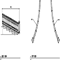Otterwood2 Extrados1 Cove1 Stair Helical NewelPier X = 46" Y = 84"Z = 9-1/2" XX = n/a YY = n/aZZ = n/a R = 76-3/4" D = n/aP = n/a \$ 2726.56Otterwood2 Straight Stairs Stair Straight Straight X = 36-5/8" Y = 12"Z = 9-1/2" XX = n/a YY = n/aZZ = n/a R = n/a D = n/aP = n/a \$ 82.00Otwell Duran Stairs Stair Straight X = 36" Y = 12"Z = 10" XX = n/a YY = n/aZZ = n/a R = n/a D = n/aP = n/a \$ 88.00Oxford Straight Stairs Stair Straight X = 36" Y = 12"Z = 9" XX = n/a YY = n/aZZ = n/a R = n/a D = n/aP = n/a \$ 106.00Verona1 Belleville1 Extrados1 Stair Spiral NewelPier X = 34" Y = 98-9/16"Z = 61" XX = n/a YY = 125-5/8"ZZ = 83" R = 300" D = n/aP = n/a \$ 999999.00